NOTE: I initially created this site while still at University, and stopped updating it several years ago. I'm leaving it here as most of the stuff here is probably still true, but please note that most is almost certainly out of date.

I've started making an up-to-date CV at cv.dalelane.co.uk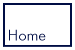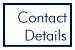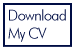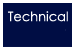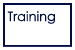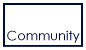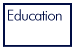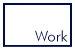Technical Software Innovation IBM Motorola University project Degree Final Year Modules Industrial Placement Second Year Modules First Year Modules Specification Transcript Web Design Bayer
 Personal Links My Links My Photos My Blog SEARCH
Technical :: Degree > First Year Modules
This page contains more detailed information about the modules I took during the first year of my Computing degree.
Aims
To provide students undertaking any type of degree study with an introductory knowledge of accounting and finance
Content
• The role of the accountant, corporate treasurer and financial controller
• Sources and uses of capital funds
• Understanding the construction and nature of the balance sheet and profit and loss account
• Principles underlying the requirements for the publication of company accounts
• Interpretation of accounts - published and internal, including financial ratio analysis
• Planning for profits, cash flow.
• Liquidity, capital expenditure and capital finance
• Developing the business plan and annual budgeting
• Estimating the cost of products, services and activities and their relationship to price.
• Analysis of costs and cost behaviour.
Aims
Information Technology (IT) is rapidly achieving ubiquity in the workplace. All areas of the business community are achieving expansion in IT and investing huge sums of money in this area. The strategic nature of technology also means that managing IT has become a core competence for modern organisations and is therefore an important part of the task of general and functional managers.
This course aims to equip students with IT management skills for the workplace. By this, we refer to those attributes that they will need to make appropriate use of IT as general or functional managers in an information-based age.
Content
Following on from the learning aims and objectives, the course is divided into two main parts: Part I considers why IT is strategic and how it can affect the competitive environment, taking stock of the opportunities and problems it provides. It consists of lectures, discussion, case studies. The objective is to investigate the business impact of IS.
Part II examines a variety of technologies available to the manager and examines how they have been used in organisations. A number of problem-oriented case studies will be given to project groups to examine and discuss. The results may then be presented in class, and are open for debate.
In summary, the aim of the course is to provide the knowledge from which students should be able to make appropriate use of computing and information technology in forthcoming careers. This necessitates some technical understanding of computing, but not at an advanced level.
Aims
To introduce the concepts of logic that underlie all mathematical reasoning and the notions of set theory that provide a rigorous foundation for mathematics.
Learning Objectives
By the end of this course, the students will be able to: understand and work with a formal definition; determine whether straight-forward definitions of particular mappings etc. are correct; determine whether straight-forward operations are, or are not, commutative; read and understand fairly complicated statements expressing, with the use of quantifiers, convergence properties of sequences.
Content
Logic:
• Definitions and Axioms
• Predicates and relations
• Logical equivalence and logical consequence
• Direct and indirect methods of proof
• Proof by contradiction
• Counter-examples
• Analysis of statements using Semantic Tableaux
• Definitions of proof and deduction
Sets and Functions:
• Sets
• Cardinality of finite sets
• Countability and uncountability
• Maxima and minima of finite sets
• Unions, intersections, and/or statements and de Morgan's laws
• Functions as rules, domain, co-domain, image
• Injective (1-1), surjective (onto), bijective (1-1, onto) functions
• Permutations as bijections
• Functions and de Morgan's laws
• Inverse functions and inverse images of sets
• Relations and equivalence relations
• Arithmetic mod p.
Sequences:
• Definition and numerous examples
• Convergent sequences and their manipulation
• Arithmetic of limits
Aims
To introduce functional programming while drawing out the similarities with abstract mathematics.
To show that the mathematical thought process is a natural one for programming.
To provide a gentle introduction to practical functional programming.
Objectives
Students should be able to write simple functions, to understand the nature of types and to use data types appropriately. They should also appreciate the value and use of recursion.
Content
Expressions, choice, scope and extent, functions, recursion, recursive datatypes, higher-order objects.
Aims
To introduce students to the basic principles of computer operation and architecture.
Objectives
Familiarity with the von Neumann model, the nature and function of each of the components, and general principles of operation of the machines, including input and output transfers and basic numeric manipulations.
Content
• Brief historical introduction to computing machines
• Binary basis of computer operation and binary numeration systems
• Von Neumann computers and the structure, nature and relationship of their major elements
• Principles of operation of digital computers
• Use of registers and the instruction cycle
• Simple addressing concepts
• programming
• Integers and floating point numbers
• Basic principles and mechanisms of data transfer
• Programmed and data channel transfers
• Device status
• Interrupt programming
• Buffering
• devices
Aims
To ensure students appreciate the concept of an algorithm as an effective procedure.
To introduce criteria by which algorithms may be chosen, and to demonstrate non-obvious algorithms.
To provide practical skills at reading and writing programs in ISO Standard C.
Objectives
Students should be able to determine the time and space complexity of short algorithms, and know 3 sorting algorithms and 2 searching algorithms.
Students should be able to design, construct and test short programs in C, using standard libraries as appropriate. They should be able to read and comprehend the behaviour of programs written by others.

To provide practical skills in low-level programming and basic computer services
Content
Algorithms:
• Introduction: Definition of an algorithm and characteristics of them.
• Basic Complexity: The efficiency of different algorithmic solutions.
• Best, average and worst case complexity in time and space.
• Fundamental Algorithms: Sorting and Searching.
• Space-time trade-offs.
• Graphs.
• Dijkstra's shortest path.
C Programming:
• Introduction: C as a simplified programming language
• ISO Standards
• Basic Concepts: Functions, variables, weak typing.
• Statements and expressions.
• Data Structuring: Enumeration, struct and arrays.
• Pointers and construction of complex structures.
• The preprocessor: #include, #if and #define
Programming:
• Input-output.
• Use of standard libraries.
• Multiple file programs.
• User interfaces.
• Professionalism: Coding standards, defensive programming, documentation, testing.
• Ethics.
Aims
To introduce students to the structure, basic design, operation and programming of conventional, von Neumann and non-von Neumann computers at the machine level.
To explore the correspondence between high level programming language control and data structures and what happens at the machine level.
Objectives
To demonstrate an understanding of how the forms and conventions of high level languages are related to the machine level.
To experience how structured programming can be applied in low as well as high level languages.
To be able to assess the potential advantages and disadvantages of different architectures.
Content
• Principles of digital computer operation: use of registers and the instruction cycle; simple addressing concepts; Integers and floating point numbers.
• Input and output.
• Introduction to digital logic.
• Aspects of modern computer architectures: Von Neumann and Non von Neumann architectures and modern approaches to machine design, including, for example, RISC (vs CISC) architectures.
• Topics in contemporary machine design, such as pipelining; parallel processing and multiprocessors.
• The interaction between hardware and software.
• Writing short assembler-level programs.
Aims and Learning Objectives
To provide students with a basic introduction to the mathematical skills necessary to tackle process engineering design applications.
Content
• Differentiation, integration
• Revision of differentiation of logarithmic, exponential and inverse trignometrical functions
• Revision of applications of integration including polar and parametric co-ordinates
• Further calculus: Hyperbolic functions, Inverse functions, McLaurin's and Taylor's Theorems, Limits, Approximate methods including the solution of equations by Newton's method and integration by Simpsons rule
• Partial differentials: Functions of several variables
• Small errors
• Total differential
• Differential equations
• Solution of first order equations using separation of variables and integrating factor
• Linear equations with constant coefficients using trial method for particular integral
• Simultaneous linear differential equations
Aims
To introduce students to the use of a workstation, to word-processing, spreadsheets and relational data bases, and to the basic ideas of computing, and to the range of applications and misapplications of computers in science.
To give students some experience of working in small groups.
Objectives
Students should have a practical ability to use contemporary information management facilities. They should be able to write a good report, and they should have the confidence and the language to enable criticism of the use of computers in science.
Content
• Introduction: hardware, software, networking
• Use of the workstation
• Social issues
• The relationship between computing and science
• Computers as calculators, as simulating engines, and as new realities
• Mathematical and computational models
• The difficulty of validating or criticising computational models
• Example of fluid flow, and the numerical wind tunnel
• Experiment and decision making using computational models
• Artificial intelligence, expert systems, neural nets, artificial evolution
• The use and abuse of computers in science
• Word processing
• HTML
• Scientific journalism and scientific reports
• The goals of succinctness and clarity
• Spreadsheets, organizing, exploring and presenting numerical data
• Introduction to Statistics: Mean, standard deviation, histograms, the idea of probability density functions
Aims
To introduce students to the use of a workstation, to wordprocessing, spreadsheets and relational databases, and to the basic ideas of computing, and to the range of applications and misapplications of computers in science.
To give students some experience of working in small groups.
Objectives
Students should have a practical ability to use contemporary information management facilities. They should be able to write a good report, and they should have the confidence and the language to enable criticism of the use of computers in science.
Content
• Normal and Poisson distributions
• A simple introduction to confidence intervals and hypothesis testing
• Elementary tools for dealing with non-normal data
• An introduction to correlation
• Computational experiments
• Databases
• Notations of set theory
• Data types and structures
• Hierarchical, network, and relational databases
• Some natural operations on relations: union, projection, selection, Cartesian product, set difference
• Design of relational databases
• Access as an example of a database system
• The integrated use of word processing, spreadsheets and relational databases

Last updated: 6 Nov 2005

Site design: Dale Lane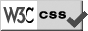Technical :: Degree > First Year Modules